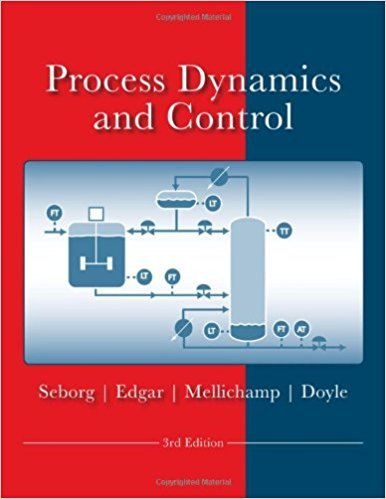×
Get Full Access to Process Dynamics And Control - 3 Edition - Chapter 18 - Problem 18.15
Get Full Access to Process Dynamics And Control - 3 Edition - Chapter 18 - Problem 18.15

×

# A process to be controlled has two controlled variables Y1ISBN: 9780470128671 148

## Solution for problem 18.15 Chapter 18

Process Dynamics and Control | 3rd Edition

• Textbook Solutions
• 2901 Step-by-step solutions solved by professors and subject experts
• Get 24/7 help from StudySoup virtual teaching assistantsProcess Dynamics and Control | 3rd Edition

4 5 1 313 Reviews
21
5
Problem 18.15

A process to be controlled has two controlled variables Y1 and Y2, and three inputs that can be used as manipulated variables, U1, U2, and U3. However, it is desired to use only two of these three manipulated variables in a conventional multiloop feedback control system. Transfer functions for the process are shown below. Which multiloop control configuration will 364 Chapter 18 Multiloop and Multivariable Control result in the smallest amount of steady-state interaction between inputs and outputs? Justify your answer. Y (s) = - 3 - U (s) - 0 5 U (s) + 1 U (s) 1 2s + 1 1 (s + 1)(s + 3) 2 s2 + 3s + 2 3 2 4 Y2(s) = -10U1(s) + s + 1 Uz(s) + (s + 1)(3s + 1

Step-by-Step Solution:
Step 1 of 3

Chapter 6 - Power function y=bx^m gives a straight line when plotted on a log-log axis - Exponential function y=be^(mx) also y=b(10)^(mx) gives a straight line when plotted on a semilog plot where the y axis is logarithmic. - Exponential functions never pass through the origin - Power function can pass through origin only if M>0 - P=polyfit(x,y,n) returns n+1 terms - Least square criterion: minimizes the sum of the squares of the residuals (sort of like a line of best fit) - Polyfit is based on the least square method

Step 2 of 3

Step 3 of 3

##### ISBN: 9780470128671

Since the solution to 18.15 from 18 chapter was answered, more than 317 students have viewed the full step-by-step answer. This textbook survival guide was created for the textbook: Process Dynamics and Control, edition: 3. The answer to “A process to be controlled has two controlled variables Y1 and Y2, and three inputs that can be used as manipulated variables, U1, U2, and U3. However, it is desired to use only two of these three manipulated variables in a conventional multiloop feedback control system. Transfer functions for the process are shown below. Which multiloop control configuration will 364 Chapter 18 Multiloop and Multivariable Control result in the smallest amount of steady-state interaction between inputs and outputs? Justify your answer. Y (s) = - 3 - U (s) - 0 5 U (s) + 1 U (s) 1 2s + 1 1 (s + 1)(s + 3) 2 s2 + 3s + 2 3 2 4 Y2(s) = -10U1(s) + s + 1 Uz(s) + (s + 1)(3s + 1” is broken down into a number of easy to follow steps, and 131 words. The full step-by-step solution to problem: 18.15 from chapter: 18 was answered by , our top Engineering and Tech solution expert on 11/15/17, 04:03PM. This full solution covers the following key subjects: variables, multiloop, control, process, inputs. This expansive textbook survival guide covers 24 chapters, and 420 solutions. Process Dynamics and Control was written by and is associated to the ISBN: 9780470128671.

Unlock Textbook Solution